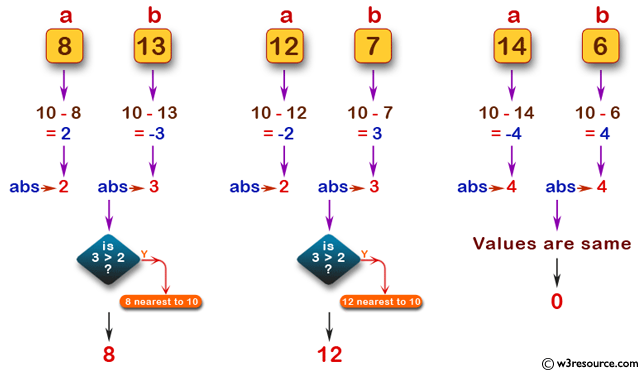﻿ Swift Basic Programming Exercise: Accept two integer values and to test which value is nearest to the value 10 - w3resource# Swift Basic Programming Exercise: Accept two integer values and to test which value is nearest to the value 10

## Swift Basic Programming: Exercise-15 with Solution

Write a Swift program that accept two integer values and to test which value is nearest to the value 10, or return 0 if both integers have same distance from 10.

Pictorial Presentation:Sample Solution:

Swift Code:

``````func close_10(_ a: Int, _ b: Int) -> Int {
if abs(10 - b) > abs(10 - a)
{
return a
}
else if abs(10 - b) < abs(10 - a)
{
return b
}
else
{
return 0
}
}
print(close_10(8, 13))
print(close_10(12, 7))
print(close_10(14, 6))
```
```

Sample Output:

```8
12
0
```

Swift Programming Code Editor:

Improve this sample solution and post your code through Disqus

What is the difficulty level of this exercise?

﻿